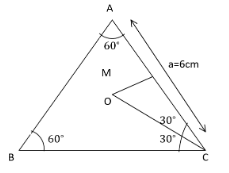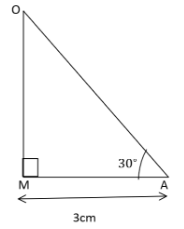QuestionAnswers

# The circumradius of an equilateral triangle whose side is $6cm$ is:A. $2\sqrt 3$ B. $3\sqrt 3$C. $4\sqrt 3$D. $5\sqrt 3$

Hint: Circumradius of an equilateral triangle is the distance from the centroid to its edges itself because for an equilateral triangle the circumcentre, orthocentre and the centroid lie at the same point which is equidistant from the edges. To find the circumradius calculate the distance of the centroid from the edge by using the diagram.

To solve this question , first of all we need to draw a rough diagram of an equilateral triangle of the side ( let it be $a$ ) $6cm$.
As all sides of the equilateral triangle are the same and all angles are of ${60^\circ }$ so we can draw the diagram as shown below.
Let $O$ be the centre and $A,B,C$ are the edges of the triangle.To calculate $OA$ we drop a perpendicular to the line $AB$. In equilateral triangle the perpendicular dropped from the centroid to the side $AB$ divides the side equally into two parts.
Therefore $AM = BM = \dfrac{{AB}}{2} = \dfrac{a}{2}$
$AM = \dfrac{6}{2} = 3cm$
Taking triangle $AOM$ into the considerationTo find $OA$ we use Cosine rule where,
Cosine$= \dfrac{{{\text{Base}}}}{{{\text{Hypotenuse}}}}$$= \dfrac{{AM}}{{OA}}$
$\cos 30^\circ = \dfrac{{3cm}}{{OA}}$ , from here we can write
As we know the value of $\operatorname{Cos} {30^\circ } = \dfrac{{\sqrt 3 }}{2}$So substituting this value in the above equation we will get $OA = \dfrac{3}{{\cos 30^\circ }} = 2\sqrt 3$ ,
Hence the value of circumradius in this case is $2\sqrt 3$.
So we can say that Option A is correct.

Note: Circumradius is the radius of the circle which surrounds a triangle. It is advised to draw a rough diagram of the triangle as it gives the idea to find the circumradius. One should know the concept that in an equilateral triangle, the circumcentre lies at the centroid.